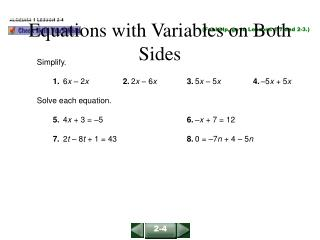DownloadDownload PresentationEquations with Variables on Both Sides

# Equations with Variables on Both Sides

Télécharger la présentation## Equations with Variables on Both Sides

- - - - - - - - - - - - - - - - - - - - - - - - - - - E N D - - - - - - - - - - - - - - - - - - - - - - - - - - -
##### Presentation Transcript

1. ALGEBRA 1 LESSON 2-4 Equations with Variables on Both Sides (For help, go to Lessons 1-7 and 2-3.) Simplify. 1. 6x – 2x2. 2x – 6x3. 5x – 5x4. –5x + 5x Solve each equation. 5. 4x + 3 = –5 6. –x + 7 = 12 7. 2t – 8t + 1 = 43 8. 0 = –7n + 4 – 5n 2-4

2. ALGEBRA 1 LESSON 2-4 Equations with Variables on Both Sides Solutions 1. 6x – 2x = (6 – 2)x = 4x2. 2x – 6x = (2 – 6)x = –4x 3. 5x – 5x = (5 – 5)x = 0x = 0 4. –5x + 5x = (–5 + 5)x = 0x = 0 5. 4x + 3 = –5 6. –x + 7 = 12 4x = –8 –x = 5 x = –2 x = –5 7. 2t – 8t + 1 = 43 8. 0 = –7n + 4 – 5n –6t + 1 = 43 0 = –12n + 4 –6t = 42 12n = 4 t = –7 n = 1 3 2-4

3. 5x – 3 – 2x = 2x + 12 – 2xSubtract 2x from each side. 3x – 3 = 12 Combine like terms. 3x – 3+ 3 = 12 + 3Add 3 to each side. 3x = 15 Simplify. = Divide each side by 3. 3x3 153 x = 5 Simplify. ALGEBRA 1 LESSON 2-4 Equations with Variables on Both Sides The measure of an angle is (5x – 3)°. Its vertical angle has a measure of (2x + 12)°. Find the value of x. 5x – 3 = 2x + 12 Vertical angles are congruent. 2-4

4. Relate: cost of plus equipment equals skateboard and equipment friend’s rental rental skateboard Define: let h = the number of hours you must skateboard Write: 60 + 1.5 h = 5.5 h ALGEBRA 1 LESSON 2-4 Equations with Variables on Both Sides You can buy a skateboard for \$60 from a friend and rent the safety equipment for \$1.50 per hour. Or you can rent all items you need for \$5.50 per hour. How many hours must you use a skateboard to justify buying your friend’s skateboard? 2-4

5. 60 + 1.5h – 1.5h = 5.5h – 1.5h Subtract 1.5h from each side. 60 = 4hCombine like terms. 604 4h4 = Divide each side by 4. 15 = hSimplify. ALGEBRA 1 LESSON 2-4 Equations with Variables on Both Sides (continued) 60 + 1.5h = 5.5h You must use your skateboard for more than 15 hours to justify buying the skateboard. 2-4

6. ALGEBRA 1 LESSON 2-4 Equations with Variables on Both Sides Solve each equation. a. –6z + 8 = z + 10 – 7z –6z + 8 = z + 10 – 7z –6z + 8 = –6z + 10 Combine like terms. –6z + 8 + 6z = –6z + 10 + 6zAdd 6z to each side. 8 = 10 Not true for any value of z! This equation has no solution b. 4 – 4y = –2(2y – 2) 4 – 4y = –2(2y – 2) 4 – 4y = –4y + 4Use the Distributive Property. 4 – 4y + 4y = –4y + 4 + 4yAdd 4y to each side. 4 = 4 Always true! The equation is true for every value of y, so the equation is an identity. 2-4